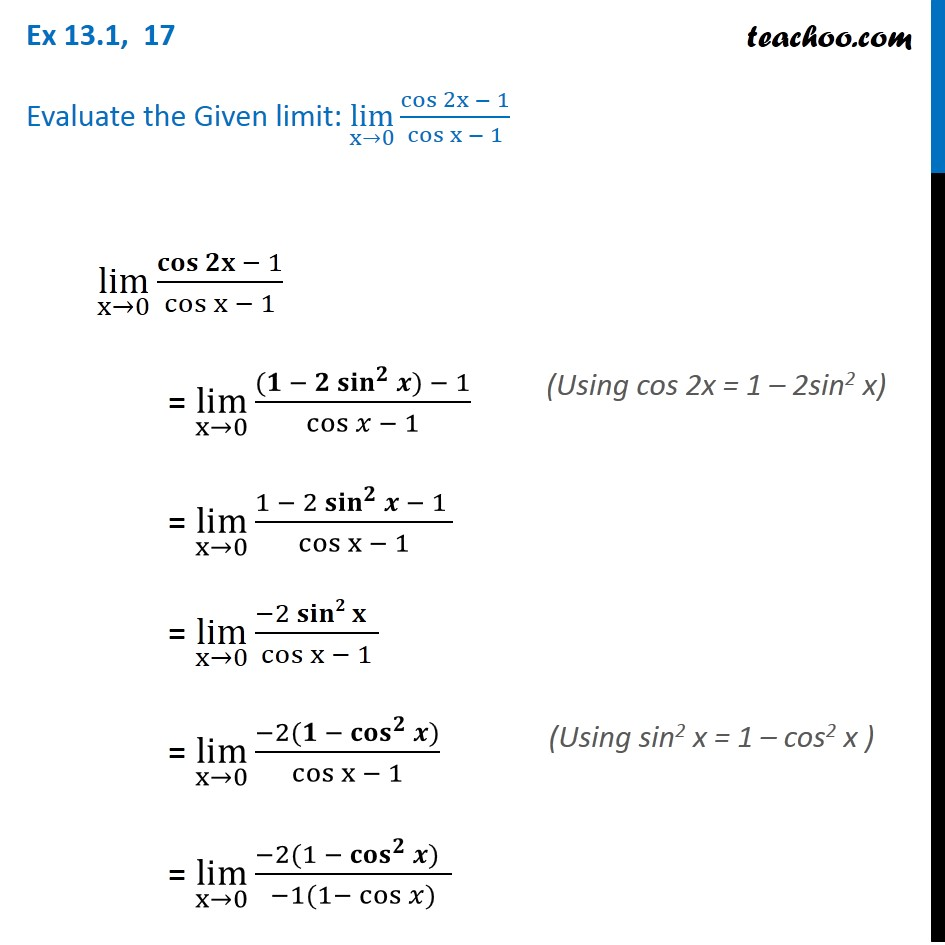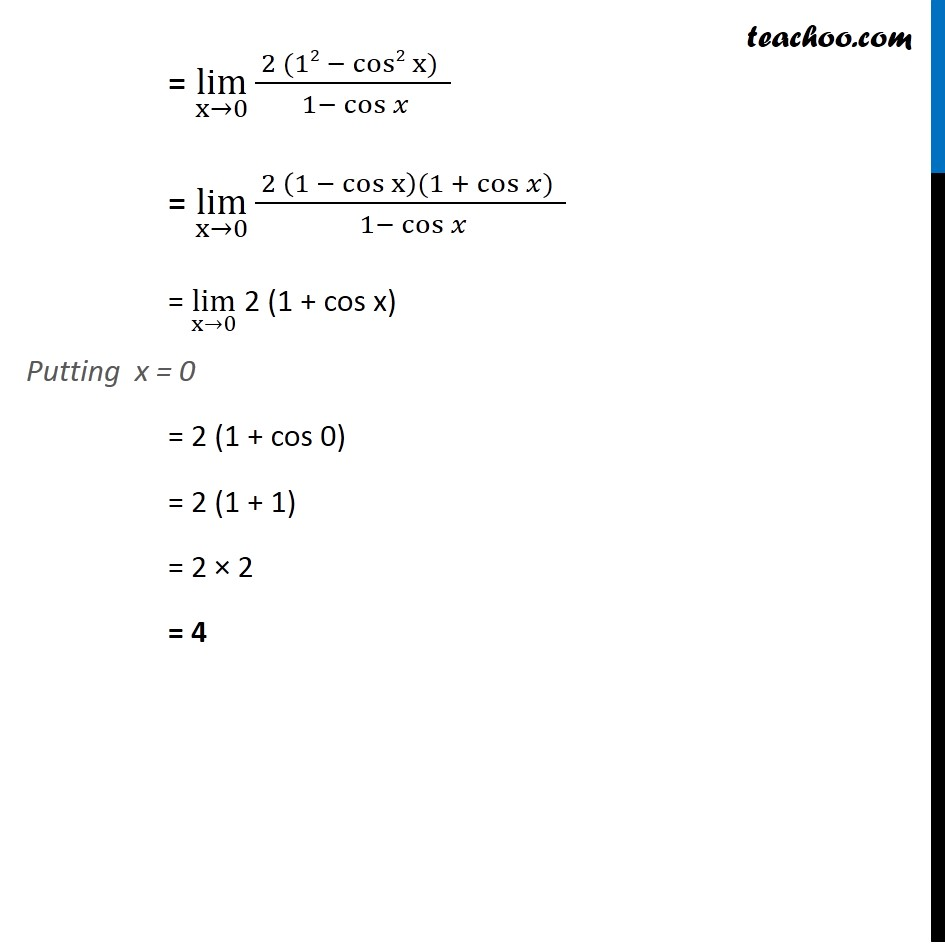Ex 13.1

Chapter 13 Class 11 Limits and Derivatives
Serial order wiseSolve all your doubts with Teachoo Black (new monthly pack available now!)

### Transcript

Ex 13.1, 17 Evaluate the Given limit: lim┬(x→0) cos⁡〖2x − 1〗/cos⁡〖x − 1〗 lim┬(x→0) ( 𝐜𝐨𝐬⁡〖𝟐𝐱 〗− 1)/cos⁡〖x − 1〗 = lim┬(x→0) ((𝟏 − 𝟐 〖𝐬𝐢𝐧^𝟐〗⁡𝒙) − 1)/cos⁡〖𝑥 − 1〗 = lim┬(x→0) (1 − 2 〖𝐬𝐢𝐧^𝟐〗⁡𝒙 − 1 )/cos⁡〖x − 1〗 = lim┬(x→0) (−2 𝐬𝐢𝐧𝟐 𝐱 )/cos⁡〖x − 1〗 = lim┬(x→0) (−2(𝟏 − 𝐜𝐨𝐬^𝟐 𝒙))/cos⁡〖x − 1〗 = lim┬(x→0) (−2(1 − 𝐜𝐨𝐬^𝟐 𝒙) )/(−1(1−〖 cos〗⁡〖𝑥)〗 ) (Using cos 2x = 1 – 2sin2 x) (Using sin2 x = 1 – cos2 x ) = lim┬(x→0) ( 2 (12 − cos2 x) )/( 1−〖 cos〗⁡𝑥 ) = lim┬(x→0) ( 2 (1 − cos x)(1 +〖 cos〗⁡〖𝑥)〗 )/( 1−〖 cos〗⁡𝑥 ) = lim┬(x→0) 2 (1 + cos x) Putting x = 0 = 2 (1 + cos 0) = 2 (1 + 1) = 2 × 2 = 4